##### The Japanese Bonsai specialist
Direct order Contact Help / Services Newsletter# Handmade bonsai exhibition table n°5 sm

Bonsaï dai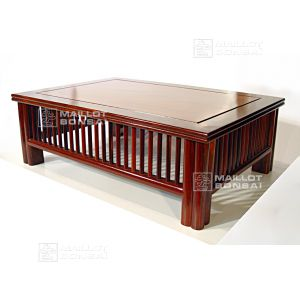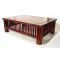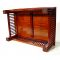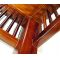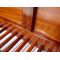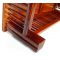ref. : 4513

360,00

Available quantity : 9Order

###### Description

Length: 60 cm. Width: 42 cm. Height: 20 cm.
The centre of the table measures 46.5*32 cm.

This classic bonsai exhibition table is typical of the stands used in Japan to present deciduous trees. The subtle panelling on the top and the Asian-style dowel rods around the edge show the skill of the woodworker. Made by hand and assembled to a high standard.

#bonsai 5.6 #table 4.5 #exhibition 4 #stands 3.6 #handmade 2.8 #woodworker 2 #assembled 1.9 #deciduous 1.9 #panelling 1.9 #standard 1.8

Formule
(( ROUND((CHAR_LENGTH(b.article_nom)-CHAR_LENGTH(REPLACE(b.article_nom, 'table', '')))/LENGTH('table')) + ROUND((CHAR_LENGTH(b.article_description)-CHAR_LENGTH(REPLACE(b.article_description, 'table', '')))/LENGTH('table')) ) * 4.5) + (( ROUND((CHAR_LENGTH(b.article_nom)-CHAR_LENGTH(REPLACE(b.article_nom, 'exhibition', '')))/LENGTH('exhibition')) + ROUND((CHAR_LENGTH(b.article_description)-CHAR_LENGTH(REPLACE(b.article_description, 'exhibition', '')))/LENGTH('exhibition')) ) * 4) + (( ROUND((CHAR_LENGTH(b.article_nom)-CHAR_LENGTH(REPLACE(b.article_nom, 'bonsai', '')))/LENGTH('bonsai')) + ROUND((CHAR_LENGTH(b.article_description)-CHAR_LENGTH(REPLACE(b.article_description, 'bonsai', '')))/LENGTH('bonsai')) ) * 3.6) + (( ROUND((CHAR_LENGTH(b.article_nom)-CHAR_LENGTH(REPLACE(b.article_nom, 'handmade', '')))/LENGTH('handmade')) + ROUND((CHAR_LENGTH(b.article_description)-CHAR_LENGTH(REPLACE(b.article_description, 'handmade', '')))/LENGTH('handmade')) ) * 2.8) + (( ROUND((CHAR_LENGTH(b.article_nom)-CHAR_LENGTH(REPLACE(b.article_nom, 'woodworker', '')))/LENGTH('woodworker')) + ROUND((CHAR_LENGTH(b.article_description)-CHAR_LENGTH(REPLACE(b.article_description, 'woodworker', '')))/LENGTH('woodworker')) ) * 2) + (( ROUND((CHAR_LENGTH(b.article_nom)-CHAR_LENGTH(REPLACE(b.article_nom, 'assembled', '')))/LENGTH('assembled')) + ROUND((CHAR_LENGTH(b.article_description)-CHAR_LENGTH(REPLACE(b.article_description, 'assembled', '')))/LENGTH('assembled')) ) * 1.9) + (( ROUND((CHAR_LENGTH(b.article_nom)-CHAR_LENGTH(REPLACE(b.article_nom, 'deciduous', '')))/LENGTH('deciduous')) + ROUND((CHAR_LENGTH(b.article_description)-CHAR_LENGTH(REPLACE(b.article_description, 'deciduous', '')))/LENGTH('deciduous')) ) * 1.9) + (( ROUND((CHAR_LENGTH(b.article_nom)-CHAR_LENGTH(REPLACE(b.article_nom, 'panelling', '')))/LENGTH('panelling')) + ROUND((CHAR_LENGTH(b.article_description)-CHAR_LENGTH(REPLACE(b.article_description, 'panelling', '')))/LENGTH('panelling')) ) * 1.9) + (( ROUND((CHAR_LENGTH(b.article_nom)-CHAR_LENGTH(REPLACE(b.article_nom, 'standard', '')))/LENGTH('standard')) + ROUND((CHAR_LENGTH(b.article_description)-CHAR_LENGTH(REPLACE(b.article_description, 'standard', '')))/LENGTH('standard')) ) * 1.8) + (( ROUND((CHAR_LENGTH(b.article_nom)-CHAR_LENGTH(REPLACE(b.article_nom, 'measures', '')))/LENGTH('measures')) + ROUND((CHAR_LENGTH(b.article_description)-CHAR_LENGTH(REPLACE(b.article_description, 'measures', '')))/LENGTH('measures')) ) * 1.8)

## Secure payment## Delivery

Our logistic partners :